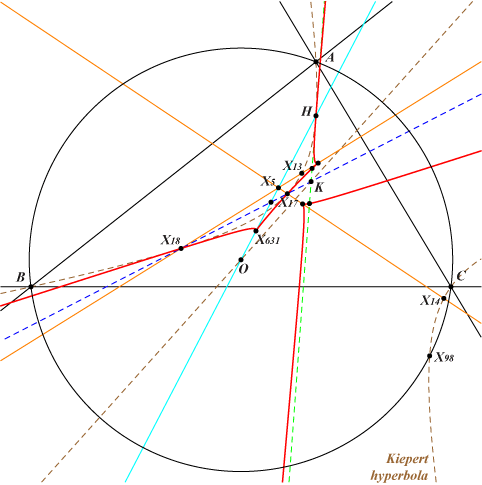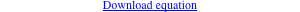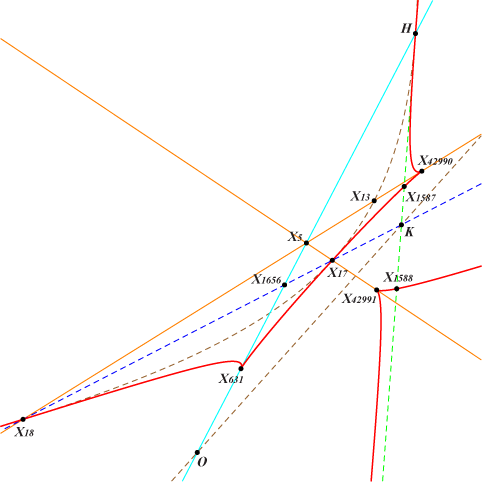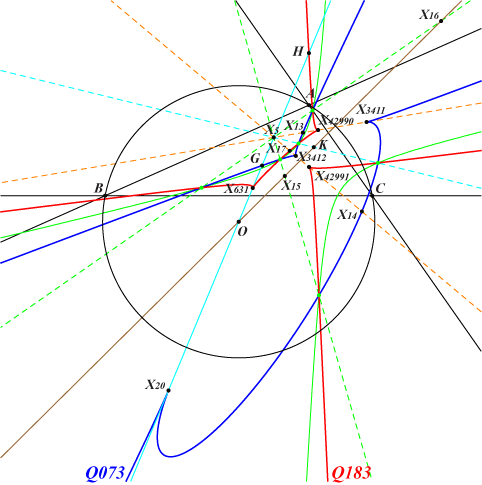too complicated to be written here. Click on the link to download a text file.X(4), X(17), X(18), X(631), X(1587), X(1588), X(39641), X(39642), X(42990), X(42991) X(39641), X(39642) are the imaginary foci of the Brocard ellipse i.e. common points of the Brocard axis and the Kiepert hyperbolaQ183 is a quartic analogous to Q073, related to points of Table 38, see remarks 5 and 4 respectively. Q183 is a tricuspidal quartic with cusps X(631), X(42990), X(42991) and cuspidal tangents passing through X(5). More precisely, these are the Euler line and the lines passing through X(13), X(14) respectively. Note that Q073 and Q183 share the same cuspidal tangents. Q183 is tritangent to the Kiepert hyperbola at X(4), with tangent passing through X(6), and at X(17), X(18) with tangents passing through X(140). The two remaining common points are X(39641), X(39642). *** Q183 is a KHO-curve, see K1191 and CL075 for information. Its KHO-equation is : x^4 - 6 x^2 (2 y^2 - 10 y z - z^2) - 9 z (4 y - z)^3 = 0. Recall that Q073 is also a KHO-curve with a similar KHO-equation : x^4 + 6 x^2 (22 y^2 - 14 y z + z^2) - 9 (2 y - z) (2 y + z)^3 = 0. The parametric KHO-representations of these two curves Q073 and Q183 are respectively : (72 t , -(3+t^2)^2 , 2 (-9-12 t^2+t^4)) and (72 t , (3+t^2)^2 , 4 t^2 (-3+t^2)), where t is a (real or complex) number or infinity.Q073 and Q183 meet at 16 points which lie four by four on four lines. • the imaginary points X(39641), X(39642) on the Brocard axis, each counted twice. • three groups on the three lines passing through X(5) and X(6), X(15), X(16). In each group, two points are real (green points on the figure) and two points are imaginary. The six real points lie on a same real (green) hyperbola (H) and the six imaginary points lie on a same imaginary ellipse (E). Both conics pass through X(39641), X(39642) and their (real) centers are on the line {5, 39, 114, 115, 1506, 1569, 2009, 2010, 2023, 2493, 2782, 3815, etc}. (H) and (E) belong to the pencil generated by the Kiepert hyperbola and the Brocard axis counted twice.